• 小试身手 python 输出最大值与最小值str_s = range(0, 1000) maxn = 0 minn = 60 for i in range(len(str_s)): if int(str_s[i]) > maxn: maxn = str_s[i] if int(str_s[i] ): minn = str_s[i] prin
小试身手 python 输出最大值与最小值
str_s = range(0, 1000)
maxn = 0
minn = 60
for i in range(len(str_s)):
if int(str_s[i]) > maxn:
maxn = str_s[i]
if int(str_s[i] < minn):
minn = str_s[i]
print('The largest is:%d,The min is %d' % (maxn, minn))
展开全文• 今天小编就为大家分享一篇python自定义函数实现最大值输出方法，具有很好的参考价值，希望对大家有所帮助。一起跟随小编过来看看吧
• 在本篇文章里小编给大家分享了关于Python中整数的最大值输出的实例内容，以及相关知识点，需要的朋友们学习下。
• python学习中，我们经常会遇到：编写一个程序，输入若干整数或者是在一串字符中，输出最大值（数）的问题。那么在这里，我给出了几种常见的，也是几种比较常用的方法，希望能给大家的学习带来一定的帮助。 第一种...
在python学习中，我们经常会遇到：编写一个程序，输入若干整数或者是在一串字符中，输出最大值（数）的问题。那么在这里，我给出了几种常见的，也是几种比较常用的方法，希望能给大家的学习带来一定的帮助。
第一种：
如果你是输入有限的整数的话，可以用到下面的这个函数：
a, b, c = map(int, input().split())

1、输入一个数字直接 m = int(input()) 2、输入两个数字就是 m, n = map(int, input().split()) 3、三个及三个以上就和两个的类似:
a, b, c = map(int, input().split())
a, b, c, d = map(int, input().split())

def max(*a):
m=a[o]
for x in a:
if x>m:
m=x
return m
s,d,f=map(int,input().split())print("最大值:” ,max(s,d,f))

运行结果：
34 22 36
最大值: 36

***Pen1cloced***

由于使用这个函数时，你要输入多少个数字你就必须要在前面给出相应个数参数，所以当你要输入整数个数较多时会比较不方便
第二种：
X=input().split()

这种方法是最简洁明了而且方便的，相比第一种你可以随便输入整数，没有任何限制
'''
遇到问题没人解答？小编创建了一个Python学习交流QQ群：778463939
寻找有志同道合的小伙伴，互帮互助,群里还有不错的视频学习教程和PDF电子书！
'''
def max(*a):
m=a
for x in a:
if x>m:
m=x
return m
#s,d ,f=map(int,input( ).split())
#print("最大值:",max(s,d,f))

if ___name__== '__main__':
x=input('输入若干数，空格隔开').split()
lst=[]
for i in x:
lst.append(int(i))
print(max( *lst))

运行结果：
输入若干数，空格隔开23 54 13 42 55
55

***Ren1closed***

第三种：
这种方法主要是用于判定一个字符串中最大数字，即使存在空格或者逗号之类的其它字符。
def max (*a):
m=a
for x in a:
if x>m:
m=x
return m
if __name___== '_main_ ':
a=input("输入:")
print(max(*a))

运行结果：
输入∶284213
8

***Renlcloced***

运行结果：
输入∶2 8,36
8

***Renlclosed***

结尾给大家推荐一个非常好的学习教程，希望对你学习Python有帮助！
Python爬虫案例教程推荐：更多Python视频教程-关注B站：Python学习者

2021年Python最新最全100个爬虫完整案例教程，数据分析，数据可视化，记得收藏哦


展开全文• 找出矩阵中的最大值，输出最大值及其所在行和列。考虑同时存在多个最大值得情况。 输入格式 使用input()函数输入，不需要任何提示信息。 输出格式 输出时使用'{}’.format()格式，提示信息为“最大值:,所在行:,所在...
• 关于python输入a,b,c三个值输出最大值和最小值的一些思考 今天开始学python不久的表弟让我给他讲题引发的一些思考： 题目：输入a,b,c三个值输出最大值和最小值 写法1： 为了能让他完全理解，最基本的写法 a = int...
关于python输入a,b,c三个值输出最大值和最小值的一些思考
今天开始学python不久的表弟让我给他讲题引发的一些思考：
题目：输入a,b,c三个值输出最大值和最小值
写法1：
为了能让他完全理解，最基本的写法
a = int(input("请依次输入a的值:"))
b = int(input("请依次输入b的值:"))
c = int(input("请依次输入c的值:"))
if a > b:
max_num = a
min_num = b
else:
max_num = b
min_num = a
if min_num > c:
min_num = c
if max_num < c:
max_num = c
print('最大值为 % s 最小值为 % s ' % (max_num, min_num))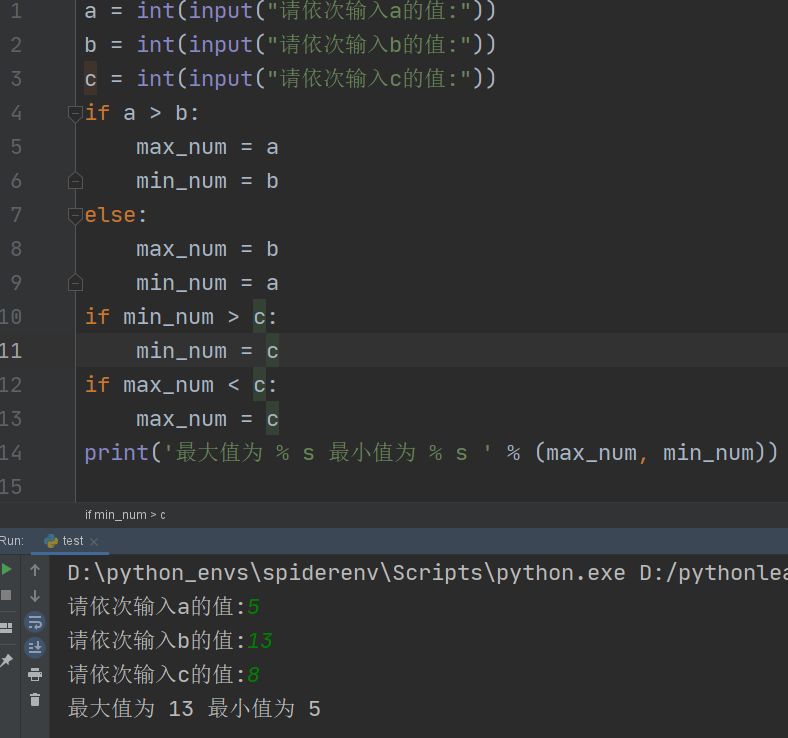写法二
他说代码太长了，能不能短一些，我就教他函数了
a = int(input("请依次输入a的值:"))
b = int(input("请依次输入b的值:"))
c = int(input("请依次输入c的值:"))
print('最大值{}, 最小值{}'.format(max(a, b, c), min(a, b, c)))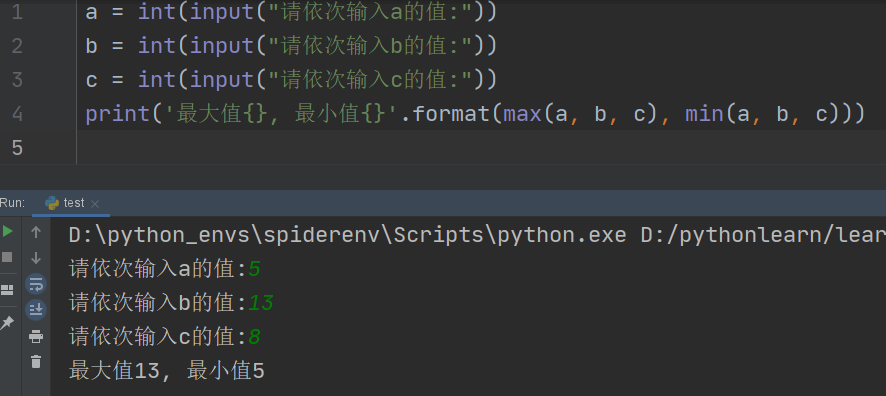写法三
他说，能不能更短一些，为了面子，我就想了一下
num = input("请依次输入a b c的值(中间用空格隔开):")
print(f'最大值为{max(list(map(int, num.split())))}，最小值为{min(list(map(int, num.split())))}')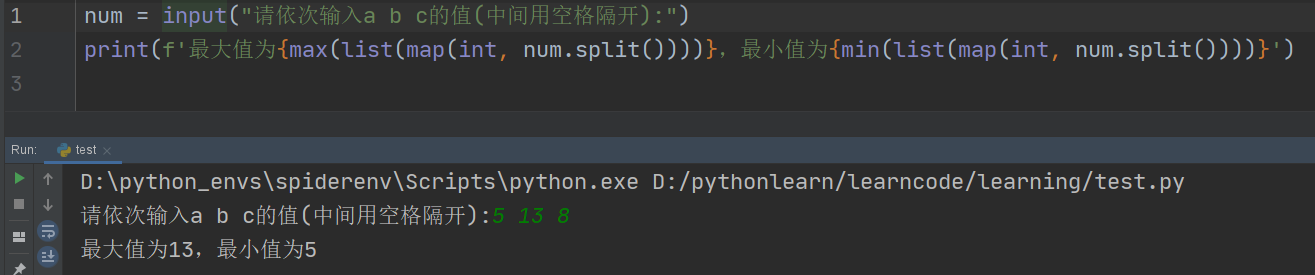总结：三种方法顺便教会了他三种格式化输出方法
最后，他说能不能一行实现，我暂时还没有想到。为了面子，只是这样胡弄他下。
num = input("请依次输入a b c的值(中间用空格隔开):"); print(f'最大值为{max(list(map(int, num.split())))}，最小值为{min(list(map(int, num.split())))}')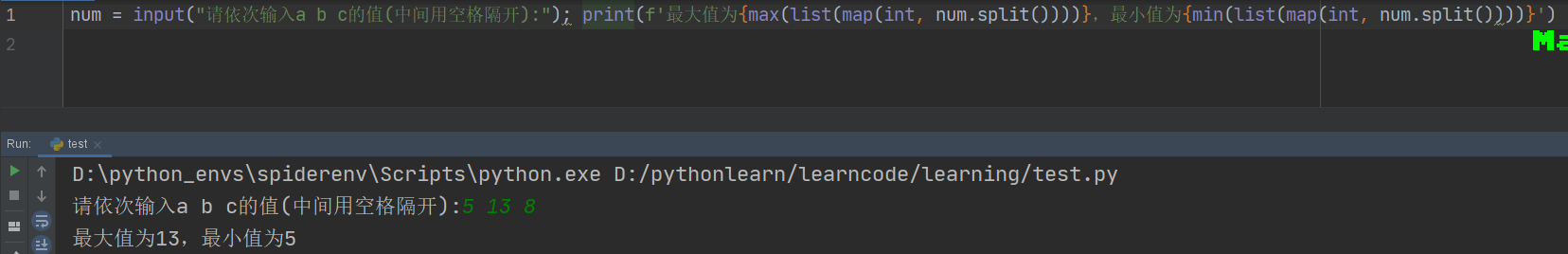补充
python高级语法
#  lambda 函数使用
a = lambda x: x + 2
print(a(2))  # 4

b = lambda x, y: x ** y
print(b(2, 3))  # 8

#  列表推导式 和 lambda 函数结合使用
li1 = [i for i in range(10) if i % 2 == 0]
print(li1)  # [0, 2, 4, 6, 8]

# 对列表内的各个元素按照某条件排序
students = [('john', 'A', 15), ('jane', 'B', 12), ('dave', 'B', 10)]
print(sorted(students, key=lambda s: s))  # 按年龄排序   [('dave', 'B', 10), ('jane', 'B', 12), ('john', 'A', 15)]

my_list = [3, 5, -4, -1, 0, -2, -6]
print(sorted(my_list, key=lambda x: abs(x)))  # 按绝对值排序  [0, -1, -2, 3, -4, 5, -6]

# 字典推导式
dic1 = {'name': i for i in range(6) if i == 4}
print(dic1)  # {'name': 4}

dic2 = {index: i for index, i in enumerate(range(6))}
print(dic2)  # {0: 0, 1: 1, 2: 2, 3: 3, 4: 4, 5: 5}

#  map  reduce filter  用法
from functools import reduce
import math

# map 对sequence中的item依次执行function(item)  然后再对可迭代对象依次进行作用
# 对列表求算术平方根
map_list = [4, 9, 16, 25, 36]
print(list(map(lambda x: math.sqrt(x), map_list)))  # [2.0, 3.0, 4.0, 5.0, 6.0]

# filter  对sequence中的item依次执行function(item)  filter然后再根据返回值True或者False决定留哪一个值
# 求1~20之间的偶数
print(list(filter(lambda x: x % 2 == 0, range(1, 21))))  # [2, 4, 6, 8, 10, 12, 14, 16, 18, 20]

# reduce function参数是一个有两个参数的函数，reduce依次从sequence中取一个元素，和上一次调用function的结果做参数再次调用function。
#  求1到5之和
print(reduce(lambda x, y: x + y, range(1, 6)))  # 15
#  上式计算原理  ((((1+2)+3)+4)+5)

# 补充zip
a = ['xiaoming', 'xiaoli', 'xiaowang']
b = [24, 25, 28]
print(list(zip(a, b)))  # [('xiaoming', 24), ('xiaoli', 25), ('xiaowang', 28)]
print(dict(zip(a, b)))  # {'xiaoming': 24, 'xiaoli': 25, 'xiaowang': 28}

dd = list(zip(a, b))
print(*zip(dd))  # (('xiaoming', 24),) (('xiaoli', 25),) (('xiaowang', 28),)


展开全文• 现在有一个二维数组test_y，我想要获取每一行的最大值，以及最大值所在索引值 代码： 随机生成一个3行4列的数组，利用np.argmax（）函数获取最大值所在索引，amax（）获取最大值的值。 import numpy as np test...
任务描述：
现在有一个二维数组test_y，我想要获取每一行的最大值，以及最大值所在索引值
代码：
随机生成一个3行4列的数组，利用np.argmax（）函数获取最大值所在索引，amax（）获取最大值的值。
import numpy as np

test_y = np.random.rand(3,4)
idx = np.argmax(test_y, axis=1)
pro = np.amax(test_y, axis=1)

print(idx)
print(pro)
运行结果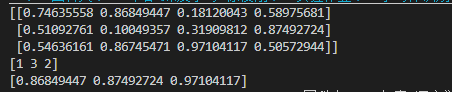展开全文• python中 .max() 函数在对 array 和 tensor 作用不同。...1. array数组使用max的效果是返回最大值 eg： zheshi = np.array([[1,2,3], [4,5,6]]) 返回： array([[1, 2, 3], [4, 5, 6]]) zheshi.max(0)...数组 tensor
• 利用python进行求解，求解的要求是不能使用python内部封装好的函数例如：max way1: def findmax(data,n): if n==1: return data else: maxi=data for i in data[1:]: if maxi&lt;i: ...max
• https://www.codenong.com/7604966/ sys.maxsize =9223372036854775807 9223372036854775807 * 7 =64,563,604,257,983,430,649 天 =181,358,438,926,919,749年 = 10亿亿年。 够了
• python版本：python3.5.1 ; IDE：pycharm2017.2 目录 一、print()函数概述 二、变量的输出 三、数据的格式化输出 ...print() 方法用于打印输出，是python中最常见的一个函数。 该函数的语法如下：...print()
• python中内置的max()函数用来得到最大值，通过冒泡排序也可以。
• 自义定函数功能，使用关键字def可定义...分别赋值给a,b,c三个数，比较大小，用if语句编写，输出最大值max def max3(a,b,c): max = 0 if a > b: if a>c: max = a else: max = c elif b>c: max = b e
• 输出：print（） print() 方法用于打印输出，最常见的一个函数。 比较运算符 >： 大于，如果运算符前面的大于后面的，则返回 True；否则返回 False < ：小于，如果运算符前面的小于后面的，则返回...数据结构
• 今天小编就为大家分享一篇Python 求数组局部最大值的实例，具有很好的参考价值，希望对大家有所帮助。一起跟随小编过来看看吧
• data = np.array([[7,6,3],[4,5,9],[7,8,9]]) 查询最大值索引的常规操作是：...2、当存在多个最大值时，argmax只会返回第一个最大值的索引，如果我全都要呢？ 自己动手写呗： import numpy as np data = np.arr....

# python输出最大值python 订阅